How to Calculate Percent Yield in Chemistry
Jun 21, 2022
Chemistry - Other

# How to Calculate Percent Yield in Chemistry

Yield is an important concept in chemistry and chemical engineering. It is defined as the amount of product obtained from a process compared to the theoretical yield.

Percent yield is used to calculate the amount of product that was actually obtained from a reaction. This information can be used to improve the reaction conditions in order to maximize the amount of product that is produced. It can also help in troubleshooting reactions that do not produce the desired product.

Learning how to calculate the percent yield in chemistry is a foundational exercise and has industrial applications. Here’s how to calculate percent yield in chemistry in 10 simple steps.

## How to Calculate Percent Yield

The theoretical yield of a reaction is the estimated amount of product that will be produced from the use of specific amounts of reactants. The percent yield is the amount of product actually yielded in comparison to the theoretical yield.

The percent yield is calculated with this mathematical formula: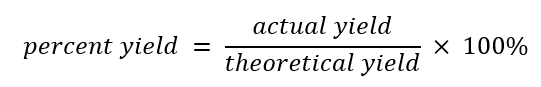The actual yield in many processes is nearly equal to the theoretical yield. In these cases, these reactions are called quantitative. In other chemical reactions, the actual yield is less than the theoretical yield, resulting in a percent yield below 100%.

The percent yield can be below 100% for a variety of reasons:

• The product of a chemical reaction is rarely found in its pure form, and some may be lost during the purification processes, lowering the yield.
• In many cases, the reactants may take part in other processes. These are known as side reactions, and the unintended by-products are called by-products. These side reactions subtract from the yield of the main product.
• In the occurrence of a reverse reaction, some of the anticipated product is likely to react with the reagents to reform the original reactants, resulting in a lower than expected yield.

The apparent yield can sometimes be greater than 100%. Because it is impossible to create something from nothing, this situation generally indicates a mistake in techniques used.

For example, in some reactions, the final product is a precipitate emerged in solvent. If the precipitate is weighed when wet with solvent, the resulting weight will be larger than anticipated. A more accurate yield measurement would require additional drying of the product.

Another explanation is that the product also contains other products from an overreaction or by-product and is contaminated. This causes the quantity of material to appear greater than anticipated. In this scenario, the product must be refined before its yield can be estimated.

## How to Calculate Percent Yield in Chemistry in 10 Steps

To learn how to calculate percent yield in chemistry, let’s review an example:

Azobenzene has the formula C6H5N and is a photoswitchable chemical. Azobenzene-based compounds are used in the textile industry as colorants and dyes.

What is the theoretical yield, percent yield, and actual yield of the following reaction if 0.10L of nitrobenzene (density = 1.20g/mL), 0.30L of triethylene glycol (density = 1.12 g/mol) yields 55g of azobenzene?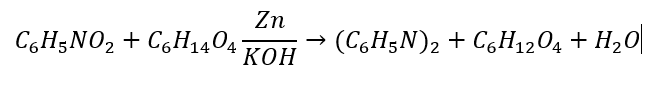### 1. Take a Balanced Chemical Equation

Ensure the chemical equation is balanced. To do that, we determine how many of each element are on each side. Then, we add coefficients until the number of each element is equal. The balanced equation is below: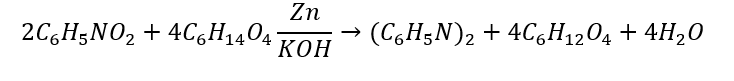### 2. Calculate Molar Mass of Reactants

Molar mass is the mass of one mole of a substance. It's also known as molar weight and can be calculated using average atomic masses on the periodic table. Use the below atomic masses taken from the periodic table to calculate the molar mass of each reactant rounded to 1 decimal point.

 Element Symbol Atomic mass Carbon C 12.0107 Hydrogen H 1.00794 Nitrogen N 14.0067 Oxygen O 15.9994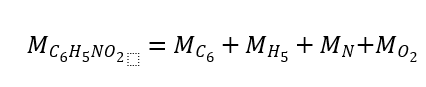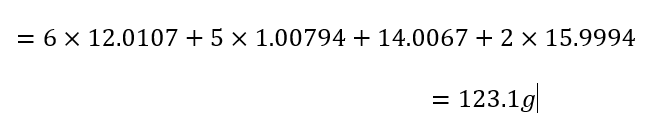And for the other reactant, triethylene glycol: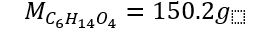Calculate the molar mass of azobenzene as well to be used in Step 7: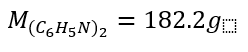### 3. Convert Reactant Amounts from Grams to Moles

We can convert from grams to moles with the formula below: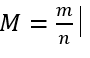Or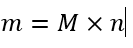Where:

• M = molar mass
• m = mass (in grams)
• n = number of moles

In the example, we have 0.10L of nitrobenzene. Convert this to moles using a line equation using the given density (g/mL).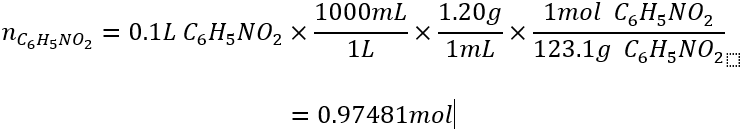We also have 0.30L of triethylene glycol. Convert this to moles with a line equation using the given density.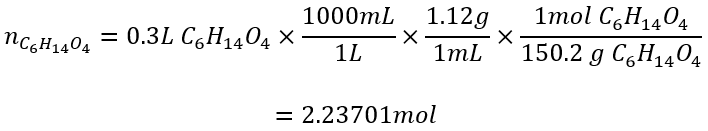### 4. Find Reaction Ratios

The stoichiometric ratios of the reactants and products can be found using the coefficients in the reaction's balanced equation. In our example we have the following balanced equation: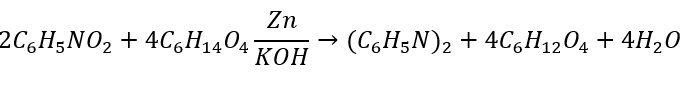The reaction ratio of azobenzene (C6H5N) and nitrobenzene (C5H5NO2) is 1:2 or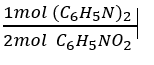Similarly, the reaction ratio of azobenzene (C6H5N) and triethylene glycol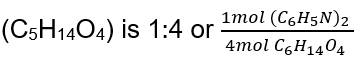### 5. Find the Ideal Ratio of Reaction

The ideal ratio of reaction refers to how many moles of each reactant are required for the desired product. In this example, 2 moles of nitrobenzene (C5H5NO2) reacts with 4 moles of triethylene glycol (C5H14O4) to produce 2 moles of azobenzene ((C6H5N)2) and 4 moles of kethoxal (C6H12O4) and 1 mole of water, also called dihydrogen monoxide (H2O).

### 6. Compare Ratios to Find the Limiting Reactant

A limiting reactant is a substance that becomes exhausted first during a chemical reaction. It's important to identify the limiting reactant in a reaction because it will determine the maximum yield that can be achieved.

In other words, knowing the limiting reactant allows you to calculate how much of a product can be produced from a given amount of starting material.

We can solve the limiting reactant problem by finding how many moles of the desired product that each of the reactants can produce. In this example, it’s: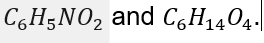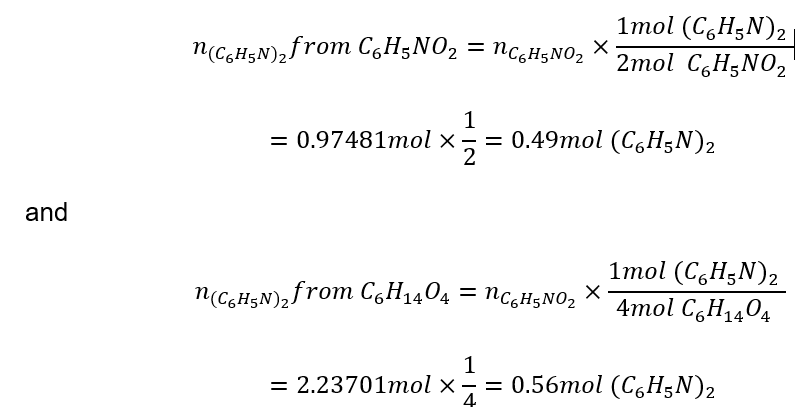In the sample problem, the limiting reactant is ni trobenzene (C5H5NO2) and as the ratio of reaction is lower, there will be excess triethylene glycol (C5H14O4).

### 7. Calculate the Theoretical Yield

The desired product in this example is azobenzene (C6H5N). Additionally, the limiting reagent in this problem is nitrobenzene (C5H5NO2). It can only theoretically produce: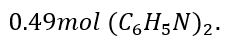.

To calculate the theoretical yield in grams, use the molar mass for azobenzene using the table in Step 2 and multiply the ratio by the number of reactant moles: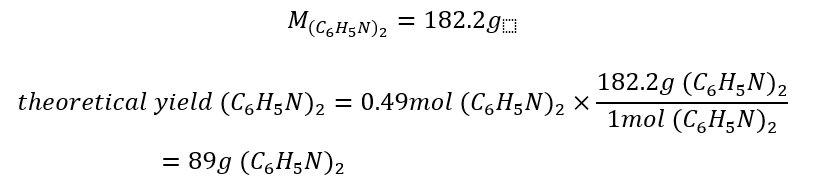### 9. Determine the Actual Yield of Experiment

The actual yield is the amount of product obtained from a chemical reaction. The actual yield is obtained experimentally. The example problem states that the actual yield is 55g of azobenzene.

When not performing an experiment, the actual yield should be provided in the problem. Be sure to convert the actual yield to grams.

### 10. Calculate the Percent Yield

Remember the difference between percent yield and theoretical yield. Percent yield is the amount of product that is produced in a chemical reaction, as opposed to the amount that was theoretically possible. To calculate percent yield, simply divide the actual yield by the theoretical yield and multiply by 100. See the following formula: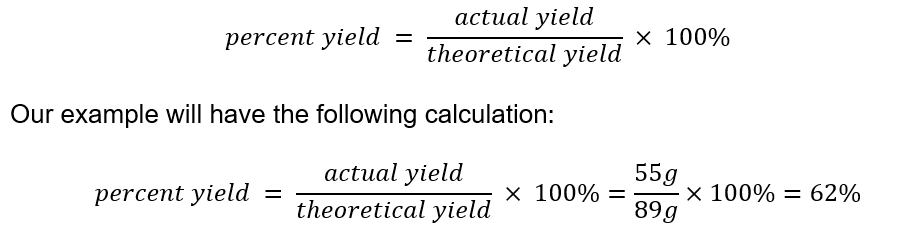## Wrapping It Up

Across the field of chemistry, it is important to be able to calculate percent yield quickly and easily. This process can be streamlined by using these simple steps.

Practice on various problems to thoroughly understand the reason behind each step in this process. This will develop your knowledge and confidence in this topic.

Don't be discouraged by a few wrong answers. Everyone makes mistakes and that's how we grow and learn. Make sure you ask for help if there is something you don't understand. Like studying for finals and writing lab reports, rote learning can seem like hard work and can be daunting. But it is vital to learning the material for test day, so keep pushing through.

View Available Chemistry Tutors24houranswers.com Parker Paradigms, Inc 5 Penn PLaza, 23rd Floor New York, NY 10001
SUBJECTS COVERED